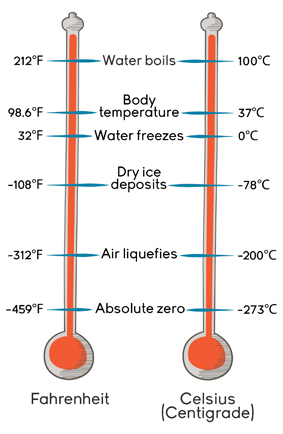Welcome to Project Lovelace! We're still in early development so there are still tons of bugs to find and improvements to make. If you have any suggestions, complaints, or comments please let us know on Discourse, Discord, or GitHub!

# Scientific temperatures

## You will learn about: submitting code and temperature conversion.

We'll get you familiar with how submitting code to Project Lovelace works by converting some temperatures. Scientists like to use Celsius instead of Fahrenheit but sometimes you're given a temperature in Fahrenheit and you have to convert it to Celsius. It's pretty easy: subtract 32 then multiply by 5/9 or if you like equations $$C = \frac{5}{9} (F - 32)$$ In the code editor try to write a function fahrenheit_to_celsius(F) that converts Fahrenheit to Celsius and we'll test your code when you submit it to make sure you got it right.Celsius and Fahrenheit are both just scales for measuring temperature. To define a scale, you just need to pick and define two temperatures. Celsius defines the 0°C to be the temperature at which water freezes, and 100°C is where water boils. Fahrenheit is older and there are different stories about how it was invented but 0°F was probably the freezing point of some ice, water and salt solution while 96°F was defined as the average human body temperature (later redefined to 98.6°F). (Image credit: Annenberg Learner )

Input: The temperature in Fahrenheit (°F).

Output: The temperature in Celsius (°C).

Example 1

Input Fahrenheit temperature: 77.9 Output Celsius temperature: 25.5

Example 2

Input Fahrenheit temperature: 32 Output Celsius temperature: 0
 Difficulty fahrenheit_to_celsius(F)

You must be logged in to view your submissions.

### Notes

• This problem is inspired by the second example in The C Programming Language.
• The Celsius and Farenheit scales read the same temperature at -40°! This is one of the test cases.
• Neither Celsius or Fahrenheit are good for science as a temperature of 0 degrees still means that some thermal energy exists. Scientists use the Kelvin scale which is 0 degrees Kelvin (K) at absolute zero (-273.15°C), the temperature at which all molecular motion ceases (but some systems still have some energy at absolute zero due to a quantum mechanical zero-point energy).
• Interestingly, the third law of thermodynamics says it's impossible to reach absolute zero.
• You can have weird physical systems with negative temperatures.
• The Celsius scale is technically defined in terms of the Kelvin scale so water actually freezes at −0.0001°C and boils at 99.9839°C (at standard temperature and pressure).
• Pretty cool list of temperature orders of magnitude.

### Videos

What the Fahrenheit?!, Veritasium
If you're interested in how the weird Fahrenheit scale came about and what it means.

Let us know what you think about this problem! Was it too hard? Difficult to understand? Also feel free to discuss the problem, ask questions, and post cool stuff on Discourse. You should be able see a discussion thread below. Would be nice if you don't post solutions in there but if you do then please organize and document your code well so others can learn from it.